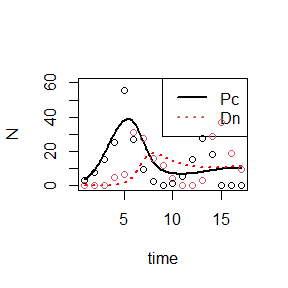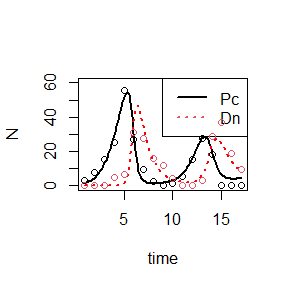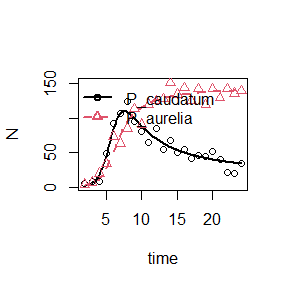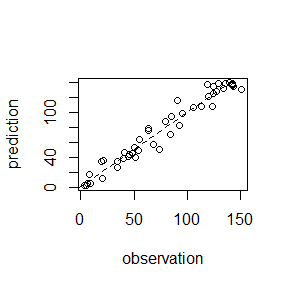# Example Analyses in gauseR

## The GauseR package

The GauseR package includes tools and data for analyzing the Gause microcosm experiments, and for fitting Lotka-Volterra models to time series data.

Below, we will demonstrate some of the basic features of this package, including several optimization methods, a function for calculating the goodness of fit for models, and an automated wrapper function. Note that the general methods applied here, as well as the form of the differential equations that we use, are described in detail in the Quantitative Ecology textbook by Lehman et al., available at http://hdl.handle.net/11299/204551. The full R package, and accompanying documentation, is available at https://github.com/adamtclark/gauseR.

## Linearized estimates

As a first example, we will use data from Gause’s experiments with Paramecium. The plotted data in the figure below shows the logistic growth of Paramecium aurelia in monoculture.

# load package
require(gauseR)
## Loading required package: gauseR
# load data
data(gause_1934_book_f22)

test_goodness_of_fit(observed = logistic_data$Volume_Species2, predicted = prediction_short) ##  0.9715139 Here, the goodness of fit is around 97%, which indicates that the model fits the data very closely. ## The optimizer As an example for using the optimizer, we use another data set of Gause’s Paramecium experiments. This predator-prey experiment shows the interaction between Didinium nasutum and P. caudatum. First, we can try to fit the model using the same three step process described above. # load data from competition experiment data(gause_1934_book_f32) # keep all data - no separate treatments exist for this experiment predatorpreydata<-gause_1934_book_f32 # get time-lagged observations for each species prey_lagged<-get_lag(x = predatorpreydata$Individuals_Prey, time = predatorpreydata$Day) predator_lagged<-get_lag(x = predatorpreydata$Individuals_Predator, time = predatorpreydata$Day) # calculate per-capita growth rates prey_dNNdt<-percap_growth(x = prey_lagged$x, laggedx = prey_lagged$laggedx, dt = prey_lagged$dt)
predator_dNNdt<-percap_growth(x = predator_lagged$x, laggedx = predator_lagged$laggedx, dt = predator_lagged$dt) # fit linear models to dNNdt, based on average # abundances between current and lagged time steps prey_mod_dat<-data.frame(prey_dNNdt=prey_dNNdt, prey=prey_lagged$laggedx,
predator=predator_lagged$laggedx) mod_prey<-lm(prey_dNNdt~prey+predator, data=prey_mod_dat) predator_mod_dat<-data.frame(predator_dNNdt=predator_dNNdt, predator=predator_lagged$laggedx, prey=prey_lagged$laggedx) mod_predator<-lm(predator_dNNdt~predator+prey, data=predator_mod_dat) # model summaries summary(mod_prey) ## ## Call: ## lm(formula = prey_dNNdt ~ prey + predator, data = prey_mod_dat) ## ## Residuals: ## Min 1Q Median 3Q Max ## -1.44938 -0.18313 -0.09181 0.61768 0.75852 ## ## Coefficients: ## Estimate Std. Error t value Pr(>|t|) ## (Intercept) 0.99795 0.29658 3.365 0.00718 ** ## prey -0.02061 0.01255 -1.642 0.13154 ## predator -0.06758 0.01831 -3.690 0.00417 ** ## --- ## Signif. codes: 0 '***' 0.001 '**' 0.01 '*' 0.05 '.' 0.1 ' ' 1 ## ## Residual standard error: 0.6755 on 10 degrees of freedom ## (4 observations deleted due to missingness) ## Multiple R-squared: 0.6355, Adjusted R-squared: 0.5626 ## F-statistic: 8.717 on 2 and 10 DF, p-value: 0.006436 summary(mod_predator) ## ## Call: ## lm(formula = predator_dNNdt ~ predator + prey, data = predator_mod_dat) ## ## Residuals: ## Min 1Q Median 3Q Max ## -0.6943 -0.3762 -0.1436 0.2799 1.3008 ## ## Coefficients: ## Estimate Std. Error t value Pr(>|t|) ## (Intercept) -0.06931 0.53816 -0.129 0.9011 ## predator -0.02602 0.01965 -1.324 0.2271 ## prey 0.03895 0.01324 2.943 0.0216 * ## --- ## Signif. codes: 0 '***' 0.001 '**' 0.01 '*' 0.05 '.' 0.1 ' ' 1 ## ## Residual standard error: 0.6432 on 7 degrees of freedom ## (7 observations deleted due to missingness) ## Multiple R-squared: 0.7039, Adjusted R-squared: 0.6194 ## F-statistic: 8.322 on 2 and 7 DF, p-value: 0.01412 # extract parameters # growth rates r1 <- unname(coef(mod_prey)["(Intercept)"]) r2 <- unname(coef(mod_predator)["(Intercept)"]) # self-limitation a11 <- unname(coef(mod_prey)["prey"]) a22 <- unname(coef(mod_predator)["predator"]) # effect of Pa on Pc a12 <- unname(coef(mod_prey)["predator"]) # effect of Pc on Pa a21 <- unname(coef(mod_predator)["prey"]) # run ODE: # make parameter vector: parms <- c(r1, r2, a11, a12, a21, a22) initialN <- c(4, 0.1) out <- deSolve::ode(y=initialN, times=seq(1, 17, length=100), func=lv_interaction, parms=parms) matplot(out[,1], out[,-1], type="l", xlab="time", ylab="N", col=c("black","red"), lty=c(1,3), lwd=2, ylim=c(0, 60)) legend("topright", c("Pc", "Dn"), col=c(1,2), lwd=2, lty=c(1,3)) # now, plot in points from data points(predatorpreydata$Day, predatorpreydata$Individuals_Predator , col=2) points(predatorpreydata$Day, predatorpreydata$Individuals_Prey, col=1)Sadly, it seems that the model doesn’t fit very well in this case. The reason turns out not to be because the model itself is bad, but rather because the method that we are using for estimating parameters is subject to high error. One way to get around this problem is to use an optimizer to directly fit the predicted dynamics to the observed data. We can do this using the lv_optim function. Note that things get a bit complicated, because we need to set the sign of the parameters (i.e. positive or negative) before we conduct the analysis. This is because a model with too many positive coefficients will lead to unbounded growth, which will ultimately crash the optimizer. For this analysis, we simply take the signs for the parameters from the estimate that we got above from the linear regressions. # Data for the optimizer: # Must have a column with time steps labeled 'time', and # columns for each species in the community. opt_data<-data.frame(time=predatorpreydata$Day, Prey=predatorpreydata$Individuals_Prey, Predator=predatorpreydata$Individuals_Predator)

# Save the signs of the parameters -
# optimizer works in log space, so these
# must be specified separately
parm_signs<-sign(parms)

# parameter vector for optimizer -
# must be a vector with, first, the
# starting abundances in log space,
# and second, the parameter values,
# again in log space
pars<-c(log(initialN), log(abs(parms)))

# run optimizer
optout<-optim(par = pars, fn = lv_optim, hessian = TRUE,
opt_data=opt_data, parm_signs=parm_signs)

# extract parameter vector:
parms <- exp(optout$par[-c(1:2)])*parm_signs initialN <- exp(optout$par[1:2])

out <- deSolve::ode(y=initialN, times=seq(1, 17, length=100), func=lv_interaction, parms=parms)
matplot(out[,1], out[,-1], type="l",
xlab="time", ylab="N", col=c("black","red"), lty=c(1,3), lwd=2, ylim=c(0, 60))
legend("topright", c("Pc", "Dn"), col=c(1,2), lwd=2, lty=c(1,3))

# now, plot in points from data
points(predatorpreydata$Day, predatorpreydata$Individuals_Predator , col=2)
points(predatorpreydata$Day, predatorpreydata$Individuals_Prey, col=1)This process is a little complicated, but it seems to fit the data much better.

## The wrapper function

Finally, let’s try a simpler example, tracking competitive interactions between P. aurelia and P. caudatum. Rather than going through all the coding involved in fitting the linear models and running the optimizer, we can simply run the gause_wrapper function, which automates all of these steps.

#load competition data
data("gause_1934_science_f02_03")

#subset out data from species grown in mixture
mixturedat<-gause_1934_science_f02_03[gause_1934_science_f02_03$Treatment=="Mixture",] #extract time and species data time<-mixturedat$Day
species<-data.frame(mixturedat$Volume_Species1, mixturedat$Volume_Species2)
colnames(species)<-c("P_caudatum", "P_aurelia")

#run wrapper
gause_out<-gause_wrapper(time=time, species=species)Again, this yields a close fit between observations and model predictions.

## Further examples

Although the optimization method that we employ is very stable, there is one disadvantage. Because parameter signs are fixed, confidence intervals estimated from this procedure are not especially informative (since they by definition cannot cross zero).

To address this problem, we also include the ode_prediction function. This function takes in parameter values and returns predictions of species abundances as a single vector. This can be useful for interfacing with other optimization functions, which can be used to produce informative confidence intervals. As an example below, we use the nls function.

#load competition data
data("gause_1934_science_f02_03")

#subset out data from species grown in mixture
mixturedat<-gause_1934_science_f02_03[gause_1934_science_f02_03$Treatment=="Mixture",] #extract time and species data time<-mixturedat$Day
species<-data.frame(mixturedat$Volume_Species1, mixturedat$Volume_Species2)
colnames(species)<-c("P_caudatum", "P_aurelia")

#run wrapper
gause_out<-gause_wrapper(time=time, species=species)# number of species
N<-ncol(gause_out$rawdata)-1 # parameters pars_full<-c(gause_out$parameter_intervals$mu) # data.frame for optimization fittigdata<-data.frame(y=unlist(gause_out$rawdata[,-1]),
time=gause_out$rawdata$time,
N=N)

yest<-ode_prediction(pars_full, time=fittigdata$time, N=fittigdata$N)
plot(fittigdata\$y, yest, xlab="observation", ylab="prediction")
abline(a=0, b=1, lty=2)#example of how to apply function, using nls()
mod<-nls(y~ode_prediction(pars_full, time, N),
start = list(pars_full=pars_full),
data=fittigdata)
summary(mod)
##
## Formula: y ~ ode_prediction(pars_full, time, N)
##
## Parameters:
##             Estimate Std. Error t value Pr(>|t|)
## pars_full1  0.574824   0.682966   0.842 0.405246
## pars_full2  4.533909   3.276244   1.384 0.174472
## pars_full3  1.734873   0.400458   4.332 0.000104 ***
## pars_full4  0.810376   0.218226   3.713 0.000654 ***
## pars_full5 -0.007675   0.002237  -3.431 0.001464 **
## pars_full6 -0.010787   0.002324  -4.640 4.05e-05 ***
## pars_full7 -0.001688   0.001048  -1.611 0.115413
## pars_full8 -0.005377   0.001317  -4.084 0.000220 ***
## ---
## Signif. codes:  0 '***' 0.001 '**' 0.01 '*' 0.05 '.' 0.1 ' ' 1
##
## Residual standard error: 10.1 on 38 degrees of freedom
##
## Number of iterations to convergence: 8
## Achieved convergence tolerance: 3.162e-06

Note, however, that in some cases parameters will still lead to unbounded growth, which will crash most optimizers. Under these circumstances, users will have to be careful and creative - e.g. by setting informative priors in a Baysian analysis.